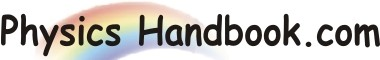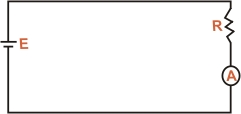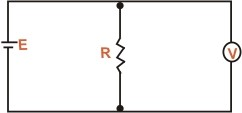HOME TOPICS DEFINITIONS TABLES LAWS INVENTIONS EXPERIMENTS QUIZ VIDEOS
 A B C D E F G H I J K L M N O P Q R S T U V W X Y Z
Ammeters and Voltmeters
The device which measure current and potential difference in circuit are called ammeters and voltmeters respectively. The basic component of these meters is the moving coil galvanometer. But before we discuss how a moving coil galvanometer is converted into an ammeter or voltmeter, let us see how these meters are connected in a circuit.
(I) Ammeter : An ammeter is connected in series with the circuit element whose current we wish to measure. It is because only then current through the circuit element also goes through the ammeter. Thus, in order to measure current through resistor R in fig (a), we place an ammeter in series with the resistor.Fig (a)
An ammeter should have a very low resistance so that on connecting it in the circuit, there is negligible change in the circuit resistance (and hence the circuit current).
(II) Voltmeter : A voltmeter is connected in parallel with the circuit component across which potential difference is to be measured. It is because potential difference (or voltmeter) refers to two points. So to measure the potential difference between two points in a circuit, we connect the two terminals of the voltmeter to those points. Thus to measure the voltmeter across resistance R fig (b), we connect the two terminals of the voltmeter to the two ends of the resistors.Fig (b)
The voltmeter should have a very high resistance so that on connecting it in the circuit, there is negligible change in the circuit resistance (and hence the circuit current).